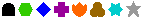Conditional Value Functions

This page describes the built-in named functions which you can use in Conditional Values. A few of these show a slightly longer form (indicated in parentheses) in the popup menu for better understanding.

lenApplied to a piece of text, this gives the number of characters in it. Applied to a number, it would give you the number of digits.

negApplied to a number, this is its negative.

notApplied to a true/false condition, this inverts the condition, true for false, false for true.

remApplied to a pair of numbers, this divides the first by the second, discards the quotient, and returns the remainder.

andApplied to a pair of true/false conditions, this is true only if both of its operand values are true, and false otherwise. Applied to a pair of numbers, this examines each binary bit of the two numbers, and returns a result that has bits =1 where both operands are 1, and zeros otherwise.

orApplied to a pair of true/false conditions, this is false only if both of its operand values are false, and true otherwise.

xorApplied to a pair of true/false conditions, this is false if they are both true or both false, and true otherwise. Applied to a pair of numbers, this examines each binary bit of the two numbers, and returns a result that has bits =0 where the bits in both operands are the same, and ones otherwise.

itm (item of)Applied to a number n and a comma-delimited text list of items, this counts out and extracts the nth item from the list.

cat (catenate)Applied to a pair of text items, this returns a new text item formed by concatenating the two together.

>=Applied to a pair of numbers or text items, this returns false if the first is less than the second, and true otherwise (greater or equal).

<=Applied to a pair of numbers or text items, this returns false if the first is greater than the second, and true otherwise (less or equal).

/=Applied to a pair of numbers or text items, this returns false if they are equal, and true otherwise (unequal).

L&NApplied to a tree node, this returns the Louw&Nida concept number of that node packed into a single number, D*1000+C.

dadApplied to a tree node, this returns the parent tree node in the tree.

broApplied to a tree node, this returns the next sibling tree node in the tree, which displays just below it in the window representation.

kidApplied to a tree node, this returns the first subtree tree node in the tree, which displays generally to the right of it in the window representation.

noun#Applied to a tree node, this returns the noun number from the ThingList, if it has one, or else null (zero).

ref (BibleRef)Applied to a tree node, this returns the Bible reference as a text string of three numbers separated by commas, book (1-66), chapter, verse, or else null if there is no verse attached to this node. Use the itm operator to extract one part.

PN?Applied to a noun number from the ThingList, this returns the pronoun number if there is a pronoun with this noun number, or else null if no pronoun refers to this Thing.

#inApplied to a tree node, this returns true (1) if the number on its left is a noun number in the tree variable on its right, not more than 9 subtrees deep, and false (null) if no such noun can be found.

L&NinApplied to a tree node, this returns true (1) if the number on its left represents a L&N concept in the tree variable on its right, not more than 9 subtrees deep, and false (null) if no such concept number can be found.

offsReturns the offset of its left operand string in its right operand string, or -1 if not there. If the right operand begins with the left operand, the value is zero (the number of other characters in front of it).

first, lastn firststr returns the first n characters from character string str; first returns the last n.

CmpTrApplied to one of the propositions in a compare relation, this returns the one role or adposition subtree from that proposition whose Thing differs from the other proposition in the relation, or null (blank) if there are more than one difference. A different adposition over the same or different noun number counts as a single difference. You can use this function to remove duplications in generating comparison text.

iconApplied to a tree node, this returns a number representing the node icon (see Tree Nodes definition):0 1 2 3 4 5 6 7

head, tailApplied to a tree list, this returns the first tree in the list, which is its head, or else the rest of the list without the first tree, which is the tail.

RelPrsApplied to a tree node, this returns a list of the relative clause subtrees under that node.

PN+Applied to a number, this returns the same number, but has the side effect that if this is used in the pronoun selection conditional, and that number is the number of a pronoun, that pronoun is activated even if no noun has been linked to it. This is the same as if the pronoun override checkbox had been checked for this pronoun, but it is effective for this instance only. If the number passed as a parameter to PN+ is greater than any pronoun, then whatever pronoun is eventually selected in this call is activated.